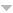# Browse & Filter

For page specific messages
For page author info
3564 records found.
Operations
Title+Summary Name Date### [NOTES/EM-03017] Potential Energy of a Dipole in External Field

Node id: 5968page

We derive expressions for force, potential energy and torque on a dipole in electric field.

23-11-02 15:11:25 n

### [NOTES/EM-04016] Boundary Condition on Interface of Two Media

Node id: 5986page

Using Stokes theorem and Maxwell's equation $$\text{curl} \vec E =0$$ it is proved that the tangential component of the electric field vanishes outside, just near the surface, vanishes.

23-11-02 10:11:41 n

### [NOTES/EM-04012] Pressure on A Charged Conducting Surface

Node id: 5941page

In this section the pressure due to a surface charge density $$\sigma$$ on  closed a conducting surface is computed and is shown to be \begin{equation*}
\text{Force per unit area}= \frac{\sigma^2}{2\epsilon_0}.
\end{equation*}

23-11-01 20:11:48 n

### [NOTES/EM-07013] Equation of Continuity and Relativity

Node id: 5919page

The equation of continuity appears in different branches of physics. It represents a local conservation law. In order to be consistent with requirement if special relativity every conserved quantity must come with a current which gives the flow of the conserved quantity across a surface and the two must obey equation of continuity. In this section we discuss these aspects of conservation laws.

23-10-31 22:10:06 n

### Optics of Moving Sources

Node id: 4290page
23-10-30 07:10:56 n

### Dispersion and Absorption of Light

Node id: 4289page
23-10-30 07:10:12 n

### Polarization of Light

Node id: 4281page
23-10-30 07:10:59 n

### Diffraction of light

Node id: 4278page
23-10-30 07:10:25 n

### Interference of light

Node id: 4277page
23-10-30 07:10:16 n

### Photometry and Geometrical Optics

Node id: 4276page
23-10-30 07:10:49 n

Node id: 1326page
• Phase velocity of an electromagnetic wave:$$v= \frac{c}{\sqrt{\varepsilon \mu}},$$ where $$c= \frac{1}{\sqrt{\varepsilon_0 \mu_0}}$$
• In a travelling electromagnetic wave:$$E\sqrt{\varepsilon \varepsilon_0} = H\sqrt{\mu \mu_0}.$$
• Space density of the energy of an electromagnetic field:$$\omega = \frac{\vec{E}.\vec{D}}{2}+\frac{\vec{B}.\vec{H}}{2}.$$
• Flow density of electromagnetic energy, the Poynting vector:$$\vec{S}=\vec{E}\times\vec{H}.$$
23-10-30 07:10:08 y

### Elastic waves, Acoustic

Node id: 1325page
• Equations of plane and spherical waves:$$\xi = a\cos(\omega t - kx), \xi = \frac{a_0}{r} \cos (\omega t - kr).$$ In the case of a homogeneous absorbing medium the factors $e^{-\gamma x}$ and $e^{-\gamma r}$ respectively appear in the formulas, where $\gamma$ is the wave damping coefficient.
• Wave equation:$$\frac{\partial^2 \xi}{\partial x^2} + \frac{\partial^2 \xi }{\partial y^2} + \frac{\partial^2 \xi}{\partial z^2} = \frac{1}{v^2}\frac{\partial^2\xi}{\partial t^2}$$
23-10-30 07:10:55 y

### Electric oscillations

Node id: 1324page

Damped oscillation in a circuit $$q=q_m e^{-\beta t} \cos(\omega t+ \alpha),$$ where $$\omega= \sqrt{ \omega ^2_0 -\beta^2, \omega_0=\frac{1}{\sqrt{LC}}}, \beta=\frac{R}{2L}.$$

23-10-30 07:10:48 y

### Mechanical oscillations

Node id: 1323page
• Harmonic motion equation and its solution:$$\ddot{x} +{\omega}^2_0 x=0, x= a\cos(\omega_0 t+ \alpha),\tag{1}$$where $\omega_0$ is the natural oscillation frequency.
• Damped oscillation equation and its solution:$$\ddot{x}+2 \beta \dot{x} +\omega^2_0 x=0, x=a_0 e^{-\beta t} \cos(\omega t + \alpha)$$ where $\beta$ is the damping coefficient, $\omega$ is the frequency of damped oscillations:$$\omega = \sqrt{\omega^2_0 - \beta^2}.$$
23-10-30 07:10:30 y

### Electromagnetic induction, Maxwell's equations

Node id: 1319page
• Faraday's law of electromagnetic induction:$$\mathscr{C}_i=-\frac{d\Phi}{di}\tag{1}$$
• In the case of a solenoid and doughnut coil:$$\Phi=N \Phi_{1} \tag{2}$$ where N is the number of turns, is the magnetic flux through each turn.
• Inductance of a solenoid:$$L=\mu \mu_0 n^2 V \tag{3}$$
• Maxwell's equations in differential form: $$\vec{\nabla} \times \vec{E} = - \frac{\partial \vec{B}}{\partial t}, \vec{\nabla}.\vec{B}=0, \\ \vec{\nabla} \times \vec{H} = \vec{j} + \frac{\partial \vec{D}}{\partial t}, \vec{\nabla}.\vec{D}=\rho,$$ where $\vec{\nabla} \times \equiv$ rot (the rotor) and $\vec{\nabla}. \equiv$ div (the divergence).
23-10-30 07:10:37 y

### Motion of charged particles in electric and magnetics fields

Node id: 1320page
• Lorentz force:$$\vec{F}=q\vec{E} + q\vec{v}\times\vec{B}.$$
• Motion equation of a relativistic particle:$$\frac{d}{dt} \frac{m_0 \vec{v}}{\sqrt{1-(\frac{v}{c})^2}}=\vec{F}.$$
• Period of revolution of a charged particle in a uniform magnetic field:$$T=\frac{2\pi m}{qB},$$ where $m$ is the relativistic mass of the particle,$$m=m_0/\sqrt{1-\left (\frac{v}{c}\right )^2}.$$
23-10-30 07:10:26 y

### Electric current

Node id: 1317page
• Ohm's law for an inhomogeneous segment of a circuit:$$I=\frac{V_{12}}{R}=\frac{\phi_{1}-\phi_{2} + \mathscr{E}_{12}}{R},$$  where $V_{12}$ is the voltage drop across the segment.
• Differential form of Ohm's law:$$\vec{j}=\sigma (\vec{E} + \vec{E}^\star),$$ where $\vec{E}^\star$ is the strength of a field produced by extraneous forces.
• Kirchhoff's laws (for an electric circuit): $$\sum I_k=0, \sum I_k R_k= \sum \mathscr{E}_k.$$
• Power P of current and thermal power Q: $$P=VI=(\phi_1 - \phi_2 +\mathscr{E}_{12})I, Q=RI^2.$$
23-10-30 07:10:42 y

### Constant magnetic field, magnetics

Node id: 1318page
• Magnetic field of a point charge $q$ moving with non-relativistic velocity $\vec{v}$: $$\vec{B}=\frac{\mu_0}{4\pi}\frac{q\, \vec{v}\times \vec{r}}{r^3}.$$
• Biot-Savart law: $$d\vec{B}=\frac{\mu_0}{4\pi} \frac{\vec{j}\times\vec{r}}{r^3}dV, d\vec{B}=\frac{\mu_0}{4\pi}\frac{I, d\vec{l}\times\vec{r}}{r^3}.$$
• Circulation of a vector $\vec{B}$ and Gauss's theorem for it: $$\oint \vec{B}.d\vec{r}=\mu_0 I, \oint \vec{B}.d\vec{S}=0.$$
• Lorentz force:$$\vec{F}=q\vec{E}+q\vec{v}\times\vec{B}.$$
• Ampere force:$$d\vec{F}=\vec{j}\times\vec{B}dV, d\vec{F}=I d\vec{I}\times\vec{B}.$$
23-10-30 07:10:19 y

### Electric capacitance energy of an electric field

Node id: 1316page
• Capacitance of a parallel-plate capacitor:$$C=\varepsilon {\varepsilon}_0 \frac{S}{d}$$
• Interaction energy of a system of point charges:$$W=\frac{1}{2}\sum q_i \phi_{i}.$$
• Energy of a charged capacitor:$$W=\frac{qV}{2}=\frac{q^2}{2C}=\frac{CV^2}{2}$$
23-10-30 07:10:44 y

### Conductors and dielectrics in an electric field

Node id: 1315page
• Electric field strength near the surface of a conductor in vacuum: $$E_n = \frac{\sigma}{{\varepsilon}_0}$$
• Flux of polarization $\vec{P}$ across a closed surface: $$\oint \vec{P}.d\vec{S}=-q^\prime,$$ where $q^\prime$ is the algebraic sum of bound charges enclosed by this surface.
• Vector $\vec{D}$ and Gauss's theorem for it: $$\vec{D}=\varepsilon \vec{E}+\vec{P}, \oint \vec{D}.d\vec{S}=q,$$ where $q$ is the algebraic sum of extraneous charges inside a closed surface.
23-10-30 07:10:20 y

X